This wacky idea occurred to me: zooming into the Mandelbrot set1 by installing a large image of it in the physical world, and just walking right up to it.

Assuming the necessary technology existed, you could print, say, a 5-meter wide image of the whole set, which would fit in one of the larger rooms of a house, and which had details fine enough to observe from as close as you could get your face to it. Your field of view would range from 5 meters wide to, say, 5 centimeters wide (to make the math easy). That’s a zoom factor of a hundred—nothing compared to the $$10^{100}$$ and greater Mandelbrot zoom videos you can find on YouTube2—but I think it might still be a cool experience. I know I enjoy closely examining a large wall map.

I think there is a relatively affordable technology for creating something like this experience: the augmented reality features built into many smart phones and tablets on the market today. On an iPhone or iPad, for example, you could render the Mandelbrot set onto a 3D rectangle, then place that rectangle into the device’s camera view of the real world using ARKit.

In a previous post, we learned how to render the Mandelbrot set onto a rectangle using Metal. But that rectangle was essentially 2D, fixed to the bounds of the MetalKit view we were using to render it. Our technique relied on that constraint to determine the complex plane coordinates of each point on the rectangle.

If we want to place our Mandelbrot rectangle anywhere in Metal’s 3D coordinate space and create a perspective view of it, we need to be able to determine the complex coordinates of each of its points regardless of how the rectangle is rotated, scaled, and translated in relation to the bounds of the view through which we’re observing it.

And we can do that, using barycentric coordinates! Recall that our rectangle is composed of two triangles. Barycentric coordinates describe each point on a triangle with three numbers that always add up to $$1$$. Each of these numbers can be interpreted as the ratio of the area of the triangle that the point makes with a pair of vertices in its parent triangle, to the area of the entire parent triangle.

This is easiest to see with an equilateral triangle.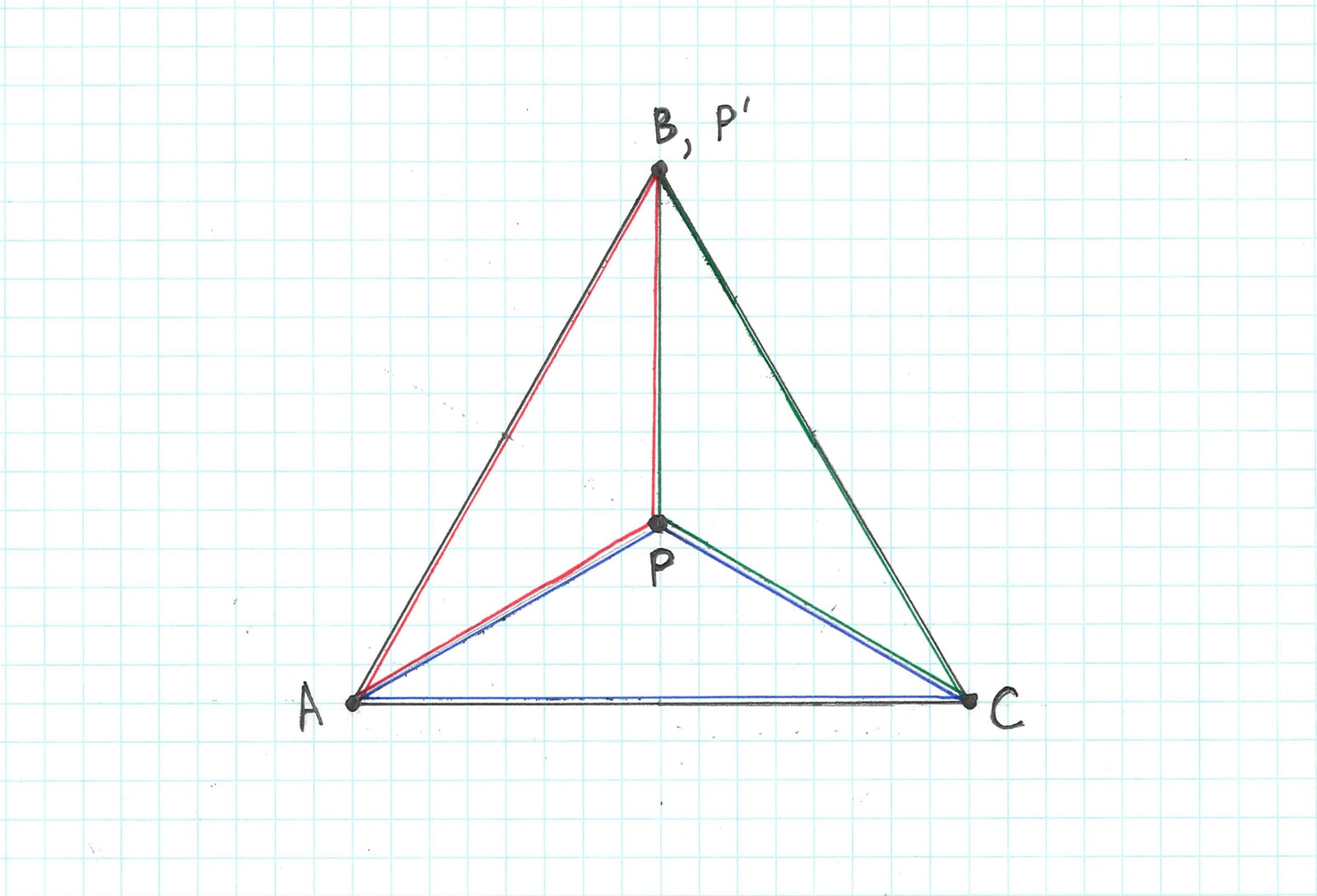For point $$P$$ in the geometric center of the triangle, the areas of triangles $$PAB$$ (outlined in red), $$PBC$$ (outlined in green), and $$PCA$$ (outlined in blue) are equal, and each is $$\frac{1}{3}$$ the area of triangle $$ABC$$, so $$P$$’s barycentric coordinates are $$(\frac{1}{3}, \frac{1}{3}, \frac{1}{3})$$.

For point $$P'$$ which coincides with $$B$$, triangle $$P'CA$$ has an area equal to that of $$ABC$$, whereas triangles $$P'AB$$ and $$P'CB$$ have zero area. $$P'$$’s barycentric coordinates are…hmmm. Are they $$(1, 0, 0)$$, $$(0, 1, 0)$$, or $$(0, 0, 1)$$? Let’s figure that out later.

Barycentric coordinates can be easily converted to rectangular coordinates. And Metal can provide the barycentric coordinates of each fragment relative to its parent triangle as arguments to a fragment shader. Just what we need to compute the complex coordinates of any point on our rectangle, regardless of how it’s positioned with respect to our view bounds.

There’s one big caveat, though: while barycentric coordinates have been available in Metal on various Macs since macOS 10.15, they are only available on the latest iOS devices based on the A14 SoC.3 I don’t have one of those, so I’m not able to jump straight to building an AR Mandelbrot experience. But I can lay the groundwork for that in a macOS playground.

Before we go too far down this path: for this project, is it necessary to render the Mandelbrot set on the GPU? Can’t we just use a pre-rendered high-resolution bitmap?

We want to simulate a print of the set that has enough detail that we can’t see pixel structure when we’re as close as we can get to it. When I point my iPhone’s camera at a ruler from as close as it can focus sharply on it, it shows about 5 cm of the ruler.4 That’s remarkably like the dimension of the set I hypothesized one could see from as close as they can get their head to it. If we want an image that has fine detail across the 1125-pixel width of an iPhone screen (to pick one example) when zoomed in a hundred times, then we’ll need a 112,500-pixel-wide image. I think that app will have an unpleasant download experience, even before you get to the unpleasant run-time experience!

Also, the Mandelbrot set looks best when certain parameters of its computation are tweaked for the current zoom level. I don’t yet have a full understanding of this phenomenon, but I think it might not even be possible to render one image of the set that looks good over a zoom range of a hundred.

I know there are lots of techniques in the 3D rendering toolbox for giving the best quality image under compute and/or memory constraints. Perhaps some of them are better applied to our problem. But I’m interested in pursuing this technique that leans heavily on compute resources. Let’s see how it goes.

We can modify our previous Mandelbrot-rendering Metal code to use barycentric coordinates. You can get the playground from GitHub if you don’t still have it lying around from working through the blog post 😉.

We need only make a few changes to the fragment shader. First, we need the fragment’s barycentric coordinates. Metal passes those in for a float3 argument annotated with [[barycentric_coord]]. Change the signature of fragment_main from

[[fragment]] float4
fragment_main(
const VertexOut in [[stage_in]],
constant ViewParams &viewParams [[buffer(1)]]
) {


to

[[fragment]] float4
fragment_main(
const VertexOut in [[stage_in]],
const float3 bc [[barycentric_coord]],
const uint primitiveId [[primitive_id]]
) {


We don’t need viewParams anymore. That’s the dependency we want to eliminate!

Note that we also ask Metal to pass in the ID of the triangle to which the fragment belongs, using the [[primitive_id]] annotation on a uint argument. Our rectangle is composed of two triangles, and we need to know which is which. This ID is the zero-based index of the triangle’s position in the vertex list5 we built with this Swift code

let positions: [simd_float4] = [
simd_float4(-1.0, 1.0, 0.0, 1.0),
simd_float4(1.0, 1.0, 0.0, 1.0),
simd_float4(-1.0, -1.0, 0.0, 1.0),
simd_float4(1.0, 1.0, 0.0, 1.0),
simd_float4(1.0, -1.0, 0.0, 1.0),
simd_float4(-1.0, -1.0, 0.0, 1.0)
]


The first three vertices describe triangle 0, and the second three describe triangle 1. Compare the vertex list with this diagram.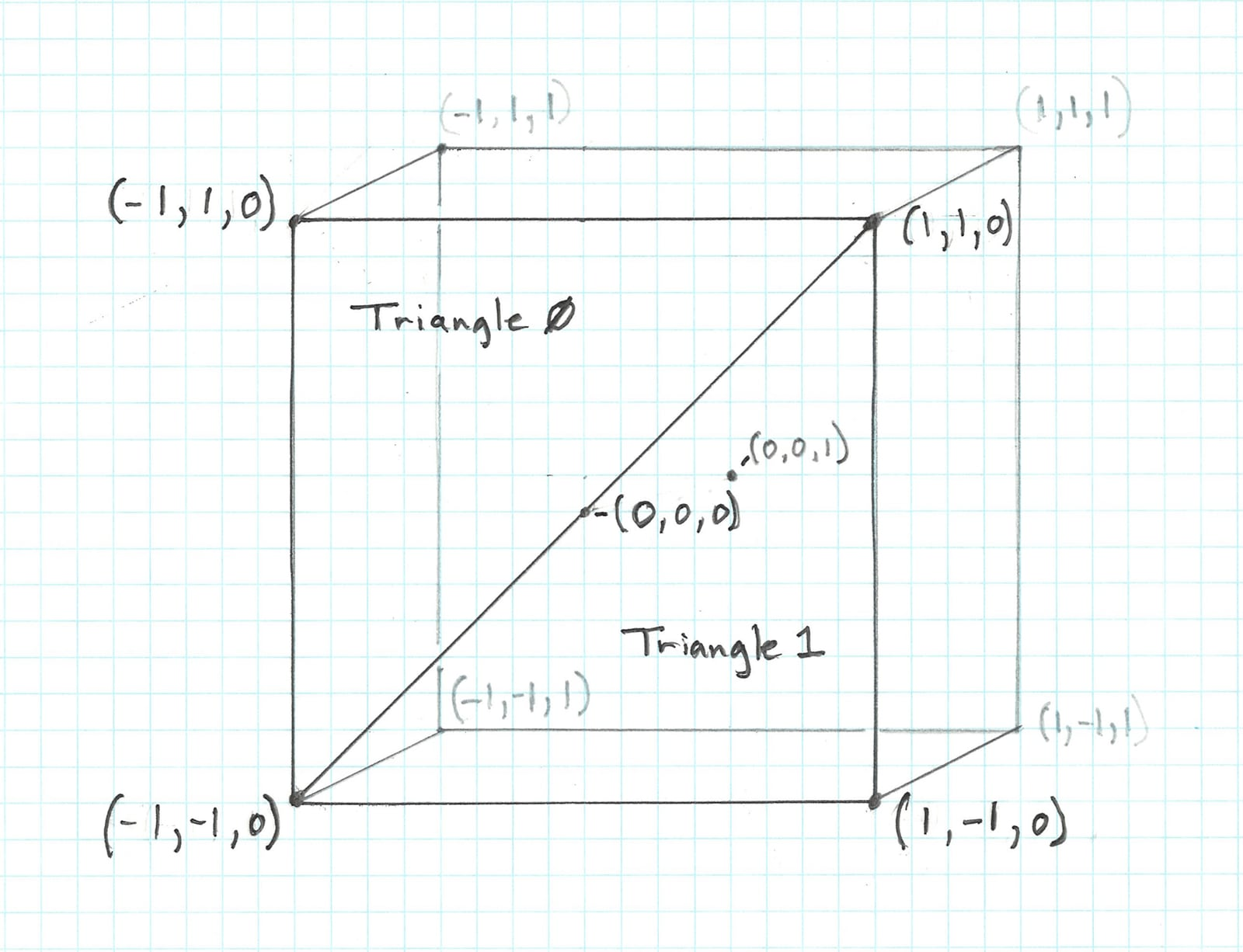How do we convert barycentric coordinates to rectangular coordinates, like those of the complex plane? The formula, which I won’t derive as I currently don’t know how to, relates the three barycentric coordinates $$\alpha$$, $$\beta$$, and $$\gamma$$6 to the rectangular coordinates of the triangle’s vertices $$A$$, $$B$$, and $$C$$ like so

\begin{align} x &= \alpha x_{A} + \beta x_{B} + \gamma x_{C} \\ y &= \alpha y_{A} + \beta y_{B} + \gamma y_{C} \end{align}

For example, the coordinates of the upper left corner of the region of the complex plane in which we want to plot the Mandelbrot set are $$(-2.0, 1.25)$$. The upper left corner happens to be the first vertex we inserted in our vertex buffer, so it corresponds with vertex $$A$$ of the triangle with index 0. We know that the barycentric coordinates of a point coinciding with a vertex are one $$1$$ and two $$0$$s, but how are they ordered? Let’s find out. Comment out the body of fragment_main and insert this new body code

  switch (primitiveId) {
case 0:
return float4(bc, bc, bc, 1);
case 1:
return float4(bc, bc, bc, 1);
}


Run the playground.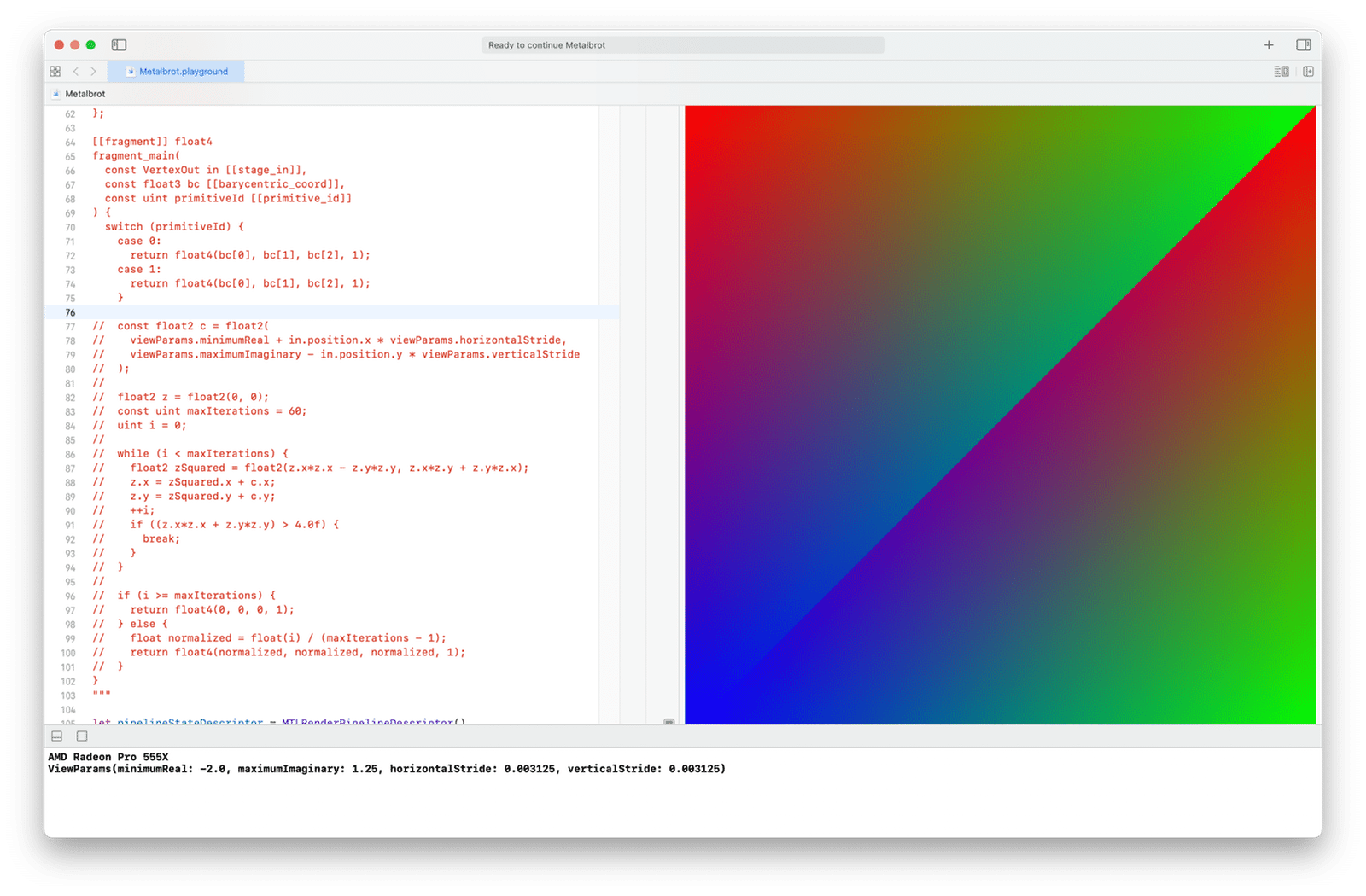We can see that the vertices of triangle 0 are colored red, green, and blue, in clockwise order, beginning with the upper left vertex, which is its first according to the vertex list ordering. Triangle 1 shows the same pattern, starting with its first vertex, the upper right. Given the code we’ve written, that implies the barycentric coordinates supplied by Metal are ordered in the same way as the triangle vertices are ordered in the vertex list. Convenient.

Now we know how to write our fragment shader using barycentric coordinates. Here it is in its entirety.7

[[fragment]] float4
fragment_main(
const VertexOut in [[stage_in]],
const float3 bc [[barycentric_coord]],
const uint primitiveId [[primitive_id]]
) {
float x, y;

switch (primitiveId) {
case 0:
x = bc * -2.0 + bc * 0.5 + bc * -2.0;
y = bc * 1.25 + bc * 1.25 + bc * -1.25;
break;
case 1:
x = bc * 0.5 + bc * 0.5 + bc * -2.0;
y = bc * 1.25 + bc * -1.25 + bc * -1.25;
break;
}

const float2 c = float2(x, y);

float2 z = float2(0, 0);
const uint maxIterations = 60;
uint i = 0;

while (i < maxIterations) {
float2 zSquared = float2(z.x*z.x - z.y*z.y, z.x*z.y + z.y*z.x);
z.x = zSquared.x + c.x;
z.y = zSquared.y + c.y;
++i;
if ((z.x*z.x + z.y*z.y) > 4.0f) {
break;
}
}

if (i >= maxIterations) {
return float4(0, 0, 0, 1);
} else {
float normalized = float(i) / (maxIterations - 1);
return float4(normalized, normalized, normalized, 1);
}
}


All that has really changed is that c’s real and imaginary components are now defined in terms of barycentric coordinates.

Run the playground.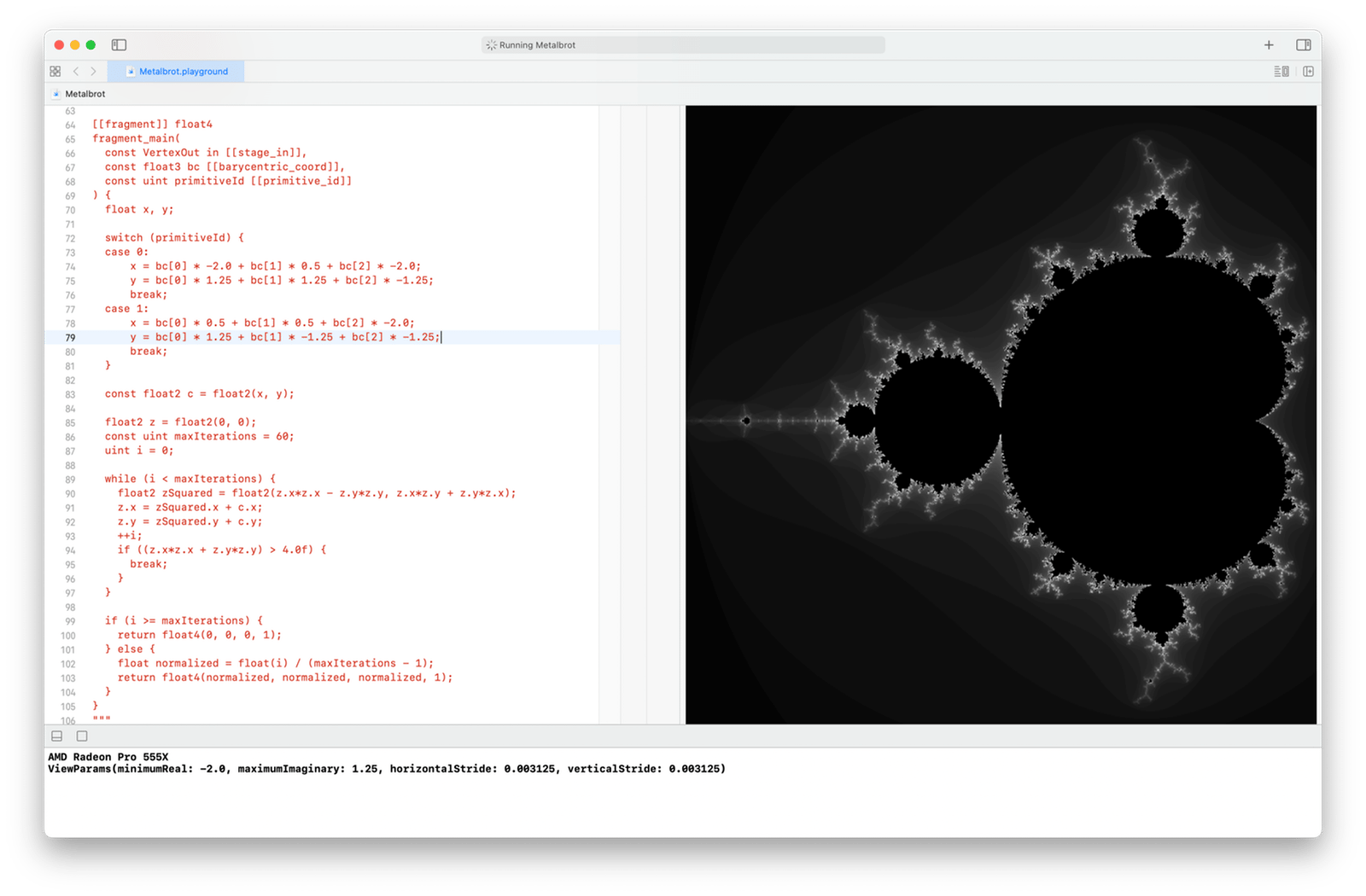It looks exactly like it did before. What have we gained? I want to explore that fully in another blog post, but here’s a teaser: we can make use of our vertex shader to transform our rectangle! For example, here’s a quick and dirty version that will rotate and skew it to give it a sense of depth.

[[vertex]] VertexOut
vertex_main(
device const float4 * const positionList [[buffer(0)]],
const uint vertexId [[vertex_id]]
) {
float rotAngle = -30.0 * M_PI_F / 180.0;
float4x4 rotation;
rotation = float4(cos(rotAngle), sin(rotAngle), 0, 0);
rotation = float4(-1.0 * sin(rotAngle), cos(rotAngle), 0, 0);
rotation = float4(0, 0, 1, 0);
rotation = float4(0, 0, 0, 1);

float4x4 skew;
skew = float4(1, 0, 0, 0);
skew = float4(0.75, 1, 0, 0);
skew = float4(0, 0, 1, 0);
skew = float4(0, 0, 0, 1);

VertexOut out {
.position = skew * rotation * positionList[vertexId],
};
return out;
}


Let’s also increase the region of the complex plane we’re plotting, so it contains the full circle of radius $$2$$ centered on the origin. That way the edges of the rectangle will blend nicely into the black background of our Metal view. Change the assignments for x and y in fragment_main.

  switch (primitiveId) {
case 0:
x = bc * -2.0 + bc * 2.0 + bc * -2.0;
y = bc * 2.0 + bc * 2.0 + bc * -2.0;
break;
case 1:
x = bc * 2.0 + bc * 2.0 + bc * -2.0;
y = bc * 2.0 + bc * -2.0 + bc * -2.0;
break;
}


Run the playground.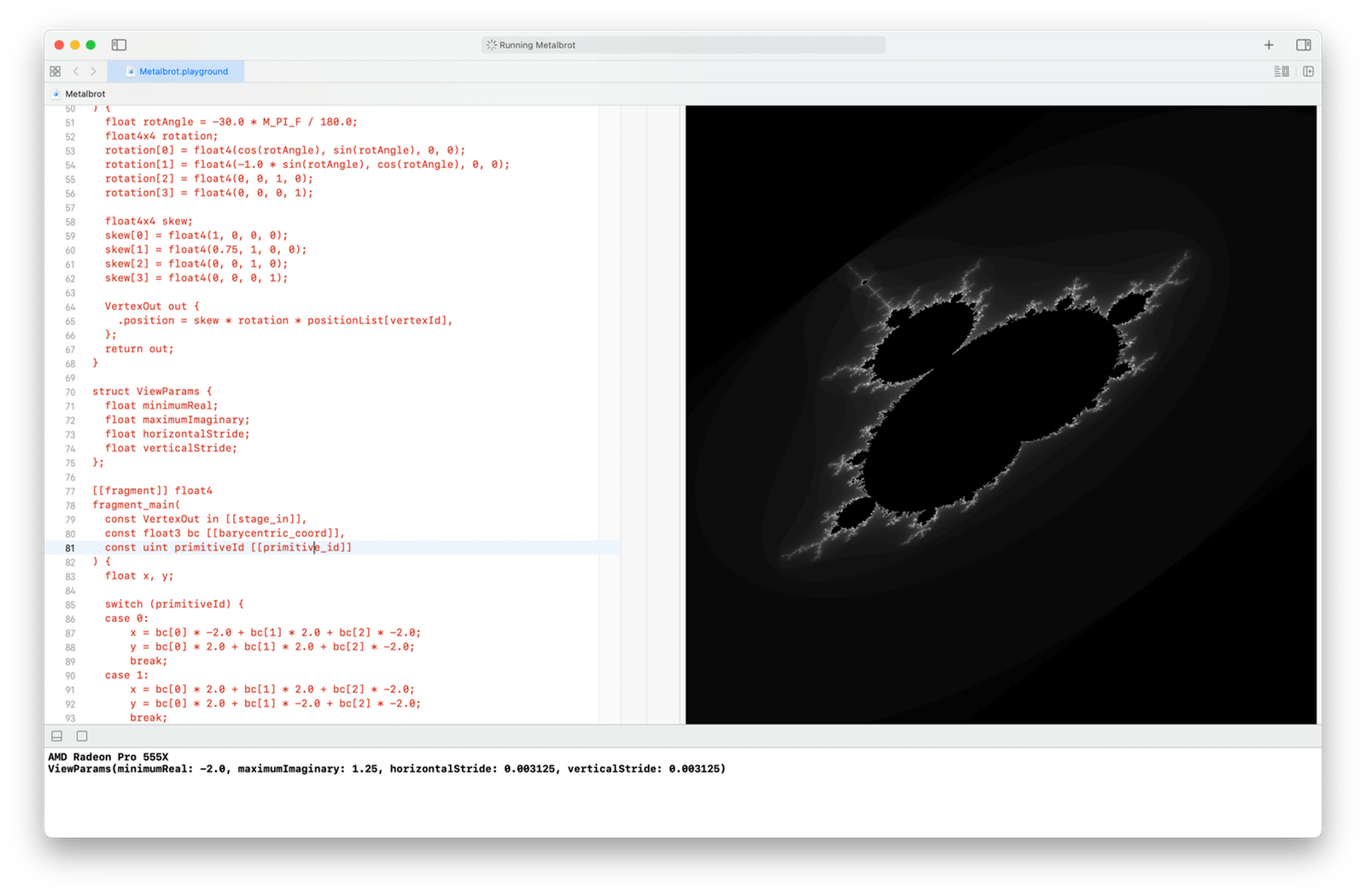Now the Mandelbrot set is flying off into spaaaace.

In the next post, we’ll develop the full set of transformations needed to render a perspective view of the set positioned in a 3D space.

1. Yeah, this is essentially a Mandelbrot set blog. What can I say? It’s been my muse this past year.

2. I couldn’t find any I liked enough to link to, but they’re there.

3. According to my reading of the Metal Feature Set Tables and the Metal Shading Language Specification

4. Roughly. Making the math easy again.

5. Two vertices are duplicated. In an app with more complex models, we’d want to eliminate vertex duplication by duplicating vertex indices instead.

6. The coordinates are given all sorts of different names in the literature. I like these.

7. This fragment function is written for pedagogy more than performance. I’d like to explore optimization opportunities in a future blog post.